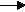gcsescience.com                                       28                                       gcsescience.com

Moles

What is Concentration?

The concentration of a solution is a measure of
the number of particles of the solute in the solvent.

A concentrated solution will have
a large number of particles of the solute in the solvent.
A dilute solution will have
a small number of particles of the solute in the solvent.

Concentrations can be expressed as
the number of moles in a particular volume or
the number of grams in a particular volume.

Moles per dm3 or mol/dm3 or mol dm-3 or
moles per cubic decimetre all mean the same thing.
A cubic decimetre is the same as a litre.

Grams per dm3 or grams/dm3 or grams dm-3
all mean the same thing.

The number of moles of solute in solution
is given by the formula

moles = (C x V) ÷ 1000

where C = concentration in mol/dm3  (moles per dm3).
V = volume in cm3.

Example 1.

In a titration, 50 cm3 of 2 mol/dm3 sodium hydroxide
was exactly neutralised by 30
cm3 of hydrochloric acid.
What is the concentration of the hydrochloric acid?

Method.
1) Write the equation for the reaction.

sodium hydroxide + hydrochloric acidsodium chloride + water.
NaOH(aq)      +           HCl(aq)NaCl(aq)    +      H2O(l)

Use the big numbers to find the proportion of NaOH to HCl.
One mole of NaOH reacts with one mole of HCl.

2) Find how many moles of sodium hydroxide are present.
moles = (C x V) ÷ 1000.

The number of moles in 50 cm3 of 2 mol/dm3 sodium hydroxide
= (2 x 50)
÷ 1000
=
0·1 moles of sodium hydroxide.

3) From 1 and 2, 30 cm3 of hydrochloric acid
also contains
0·1 moles.

You can rearrange  moles = (C x V) ÷ 1000  to give

C = (moles x 1000) ÷ V

C = (0·1 x 1000) ÷ 30
= 3·33.

The concentration of hydrochloric acid is 3·33 mol/dm3.

gcsescience.com       The Periodic Table       Index       Moles Quiz       gcsescience.com

Copyright © 2015 gcsescience.com. All Rights Reserved.